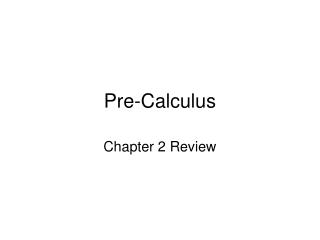DownloadDownload PresentationPre-Calculus

# Pre-Calculus

Télécharger la présentation## Pre-Calculus

- - - - - - - - - - - - - - - - - - - - - - - - - - - E N D - - - - - - - - - - - - - - - - - - - - - - - - - - -
##### Presentation Transcript

1. Pre-Calculus Chapter 2 Review

2. Vocabulary • Standard (Vertex) Form of a parabola • Vertex and Roots of a Parabola • Equation from Point and Vertex • End Behavior • Graphing Polynomials • Long Division, Synthetic Division, Remainder Theorem • Complex Number Operations • Solutions to Quadratics, Discriminant • Rational Root Theorem • Type and Number of Roots, Descartes’ Law of Signs, Bounds • Finding all complex roots • Asymptotes: (Horizontal Vertical Slant Holes) • Graphing Rational Functions • Partial Fractions

3. Answer: 4th degree polynomial Even degree polynomial 1.) Give the degree of the polynomial with the graph below:

4. Answers: 2.) 4 zeros 3.) Real zeros 2.) Give the number of zeros of that polynomial3.) Give the types of zeros of that polynomial

5. 4.) If 2i is a zero of a polynomial, what else must also be? Answer: -2i must also be a zero by the conjugate pair theorem

6. 5.) If f(2) = 4, and f(4) = -2, what must occur between x = 2 and x = 4? Answer: There must be a zero between x=2 and x=4 by the IVT

7. What are the possible numbers of positive and negative real zeros of the function? Answer: 4, 2, or 0 positive real roots 0 negative real roots What are its possible rational roots? Answer: 6.) Given the polynomial ,

8. 7.) Decompose the fraction into its partial fractions. Answer: Let x = 1 Let x = 7

9. Find an upper and lower bound for the zeros of the function Upper: x = 2 Lower: x = -6 Use synthetic division to show that 2 is a root of the function. Use the remainder theorem to show that –2 is also a root of the function. Use the quadratic formula and factoring to find the remaining zeros. 8.) Given:

10. Find the discriminant of the equation D = -16 Tell what the discriminant says about the equation. Non-real Zeros Complete the square to find the zeros of the equation. 9.) Given:

11. VA: x = 3, x = -1 HA: y = 0 VA: x = -2 Hole: (2, .25) HA: y = 0 10.) Give the asymptotes for each of the following.

12. 10.) Give the asymptotes for each of the following. VA: x = 0 HA: None SA: y = x - 2.5

13. Vertical Asymptote at x = 2 and x = -4, Horizontal Asymptote at y = 0, Hole at (3, x) No Vertical Asymptote, A Slant Asymptote, Hole at (-2, x) 11.) Give an equation for the rational function with the given properties.